## 为什么要使用表面

GM中有着绘图目标（drawing target）这一概念。绘制目标可以理解为画布，而 draw_系列函数可以理解为画笔，现实中，在一块画布上作画不会影响其他画布上的内容，同样的，在 GM 中，draw_系列函数会将图案文字等绘制在指定的绘制目标上，不会影响其他绘制目标上的内容。

1. 步结束事件
2. 清空屏幕内存
3. 绘制背景图片（到内存中）
4. 调用深度大于 1000000 的实例的绘制事件（到内存中）
5. 绘制贴图（到内存中）
6. 调用深度小于 1000000 的实例的绘制事件（到内存中）
7. 从内存中输出画面到窗口上
8. 下一帧的步开始事件

1. 其他事件
2. 清空内存
3. 绘制事件
4. 内存输出到窗口
5. 其他事件
6. ……

## 表面基础

### 创建表面

• `surface_create(w, h)` 创建一个宽为 w 像素，高为 h 像素的表面。该函数返回被创建的表面的索引（id），请务必保存好该索引。若返回 -1，则表面创建失败。注意：索引是从 0 开始的，因此，你在初始化用来储存索引的变量时，请初始化为 -1 而不是 0。

### 销毁表面

• `surface_free(id)` 销毁索引为 id 的表面。正如上所说，表面不会因为房间变换而消失或改变，因此，切记要自己销毁表面。

### 检测表面

• `surface_exists(id)` 检测索引为 id 的表面是否存在。再次强调，请将储存索引的变量初始化为 -1，因为 `surface_exists(0)` 可能是 true。

### 设置绘制目标

• `surface_set_target(id)` 将绘制目标设置到索引为 id 的表面上，此后所有 draw_系列函数均绘制到这个表面上。
• `surface_reset_target()` 将绘制目标重新设置回屏幕上。

### 绘制表面

• `draw_surface(id, x, y)` 将索引为id的表面的内容绘制在 (x, y) 的位置，表面的左上角与 (x, y) 重合。正如上所说，表面之间也可以随便转移内容，因此你可以把绘制目标设置在表面 2 上，然后 `draw_surface(表面 1, x, y)` 也是可以的。
• `draw_surface_part(id, left, top, width, height, x, y)` 将索引为 id 的表面的部分内容，左上角为 (left, top)，右下角为 (left + width, top + height) 之间的矩形部分绘制在 (x, y) 坐标处，左上角与 (x, y) 重合。

• `surface_copy(destination, x, y, source)` 除了先 `surface_set_target``draw_surface` 外，你也可以用这个函数将索引为 source 的表面内容绘制在索引为 destination 的表面的 (x, y) 处，无需改变当前绘制目标。
• `surface_copy_part(destination, x, y, source, xs, ys, ws, hs)` 同上，将索引为 source 的表面的部分内容，左上角为 (xs, ys)，右下角为 (xs + ws, ys + hs) 的矩形部分绘制在索引为 destination 的 (x, y) 坐标处，左上角与 (x, y) 重合。

### 使用表面的一般流程

```1 2 // 创建一个表面，大小根据实际需要决定。较为通用的情况是使用房间大小作为表面大小。 surf = surface_create(room_width, room_height); ```

```1 2 3 4 5 6 7 8 9 // 有时候 GM 可能会无缘无故地销毁一个表面，保险起见先判断一下。 if (!surface_exists(surf)) surf = surface_create(room_width, room_height); // 将绘制目标转移到表面上。 surface_set_target(surf); // 之后开始绘制任意东西。 draw_xxxx(); // 将绘制目标复原到屏幕。 surface_reset_target(); ```

```1 2 // 将表面绘制到屏幕上。 draw_surface(surf, 0, 0); ```

```1 2 3 // 当被销毁时释放表面 if (surface_exists(surf)) surface_free(surf); ```

## 表面高级

```1 draw_clear_alpha(c_black, 0); ```

```1 2 surface_save(id, fname); surface_save_part(id, fname, x, y, w, h); ```

```1 screen_redraw(); ```

```1 2 3 with (all) if (sprite_index != -1) drawSelf(); ```

```1 2 3 with (all) if (id != other.id && sprite_index != -1) drawSelf(); ```

```1 draw_set_blend_mode(bm_subtract); ```

`bm_subtract` 可以说是专门为表面设计的，当绘制目标为表面时，这个混色效果会给表面“挖洞”，即表面与图案重叠的部分会被清空透明。

```1 draw_set_blend_mode_ext(bm_zero, bm_src_alpha); ```

## 表面范例

### 视野光圈限制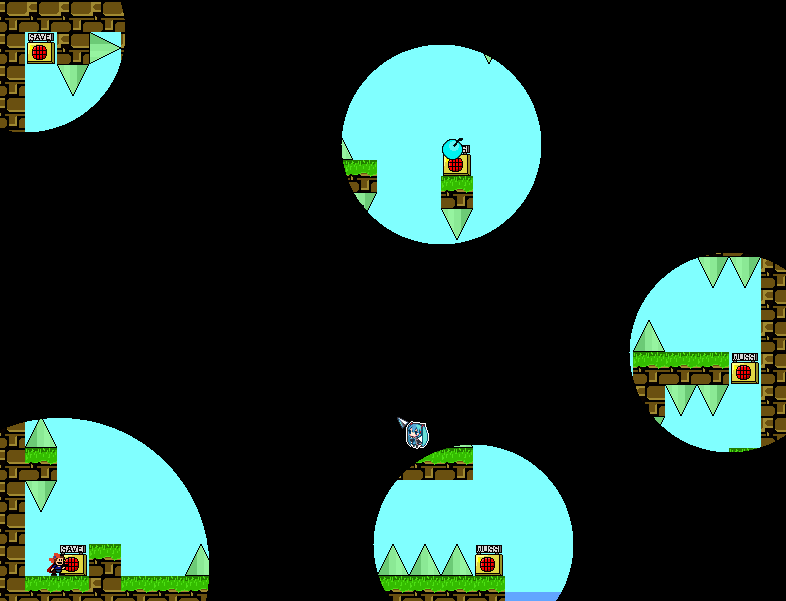Create（创建）事件中：

```1 surf = surface_create(room_width, room_height); ```

End Step（步结束）事件中：

```1 2 3 4 5 6 7 8 9 10 11 12 13 14 15 if (!surface_exists(surf)) surf = surface_create(room_width, room_height); surface_set_target(surf); draw_clear(c_black); draw_set_blend_mode(bm_subtract); // 不必是白色，是什么颜色都行，此处只是个人习惯 draw_set_color(c_white); draw_set_alpha(1); // 下面两个 with 修改为你要在周围绘制光圈的 obj，绘制圆也可以换成绘制任何图案 with (player) draw_circle(x, y, 150, 0); with (savePoint) draw_circle(x, y, 100, 0); draw_set_blend_mode(bm_normal); surface_reset_target(); ```

Draw（绘制）事件中：

```1 draw_surface(surf, 0, 0); ```

Destroy（销毁）事件中：

```1 2 if (surface_exists(surf)) surface_free(surf); ```

### 电磁波干扰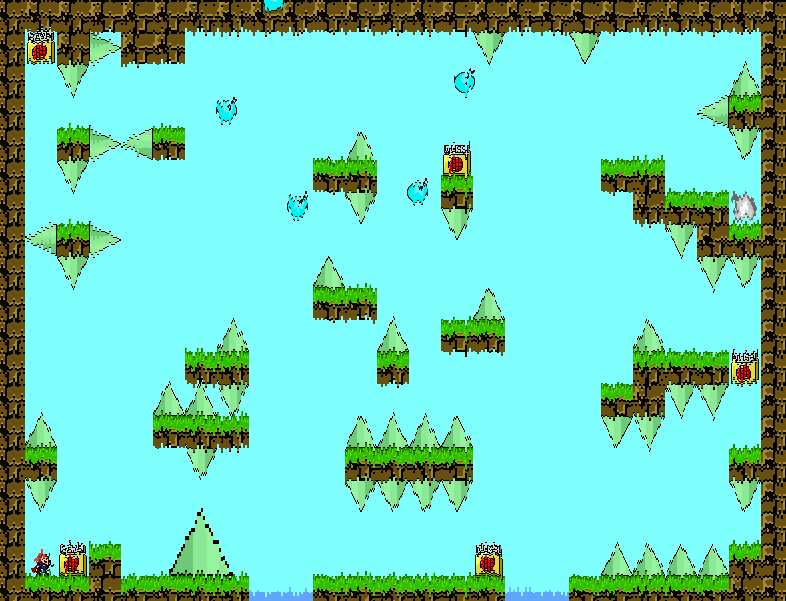Create（创建）事件：

```1 2 surf = surface_create(room_width, room_height); isRedraw = false; ```

End Step（步结束）事件：

```1 2 3 4 5 6 7 8 if (!surface_exists(surf)) surf = surface_create(room_width, room_height); surface_set_target(surf); draw_clear_alpha(c_black, 0); isRedraw = true; screen_redraw(); isRedraw = false; surface_reset_target(); ```

Draw（绘制）事件：

```1 2 3 4 5 6 7 8 var i; if (!isRedraw) { draw_set_blend_mode_ext(bm_one, bm_zero); for (i = 0;i < room_width;i += 2) draw_surface_part(surf, i * 2, 0, 2, room_height, i * 2, random_range(-5,5)); draw_set_blend_mode(bm_normal); } ```

Destroy（销毁）事件中：

```1 2 if (surface_exists(surf)) surface_free(surf); ```

```1 2 3 4 5 6 7 8 var i; if (!isRedraw) { draw_set_blend_mode_ext(bm_one, bm_zero); for (i = 0;i < room_height;i += 2) draw_surface_part(surf, 0, i * 2, room_width, 2, random_range(-5,5), i * 2); draw_set_blend_mode(bm_normal); } ```

### 化零为整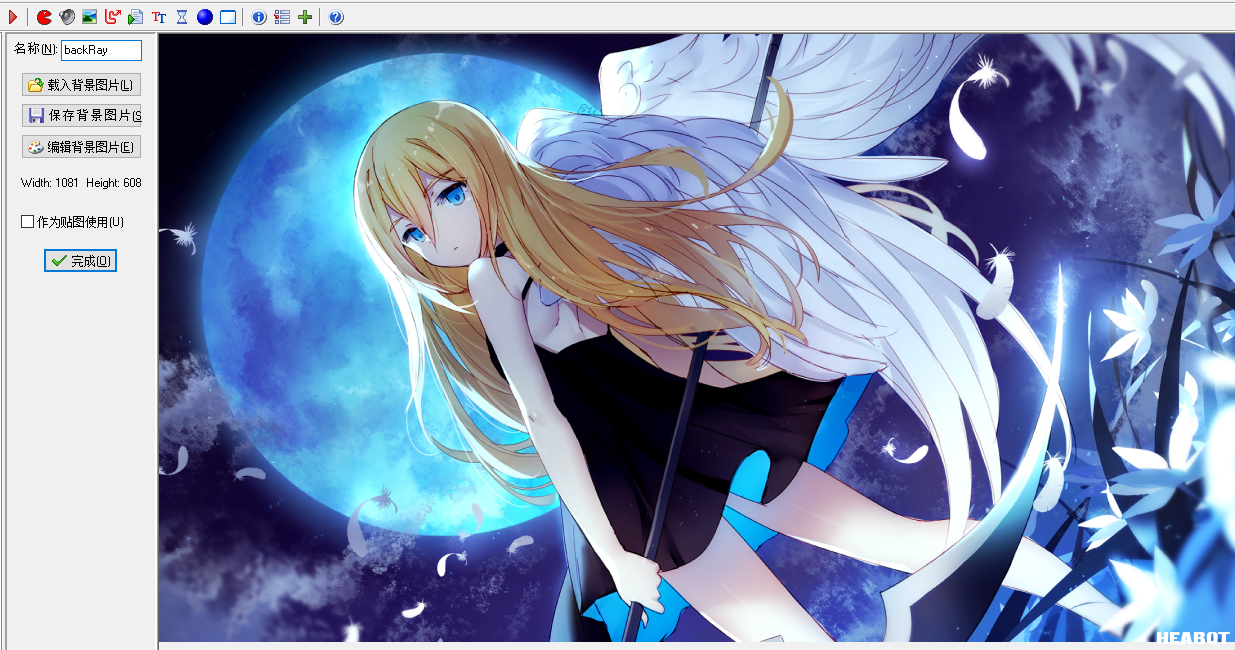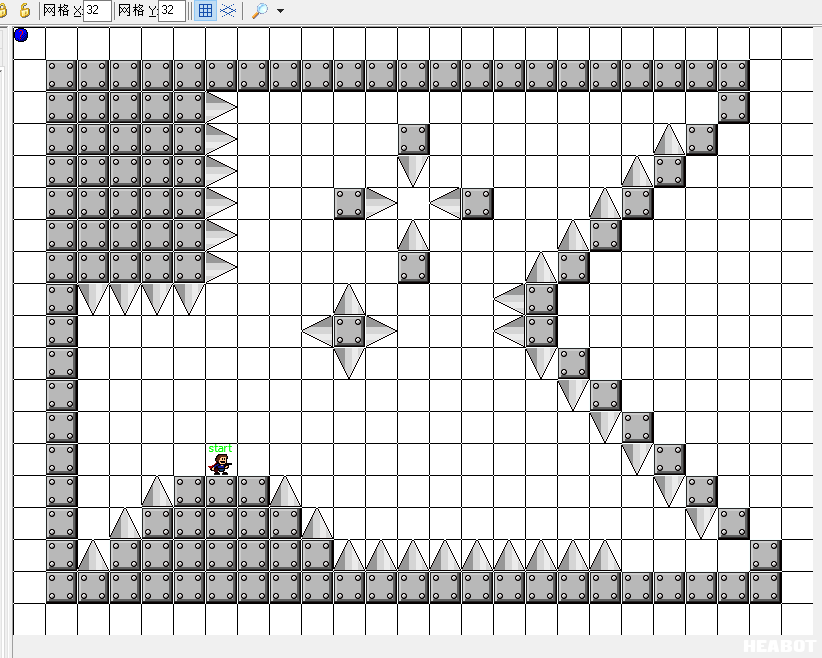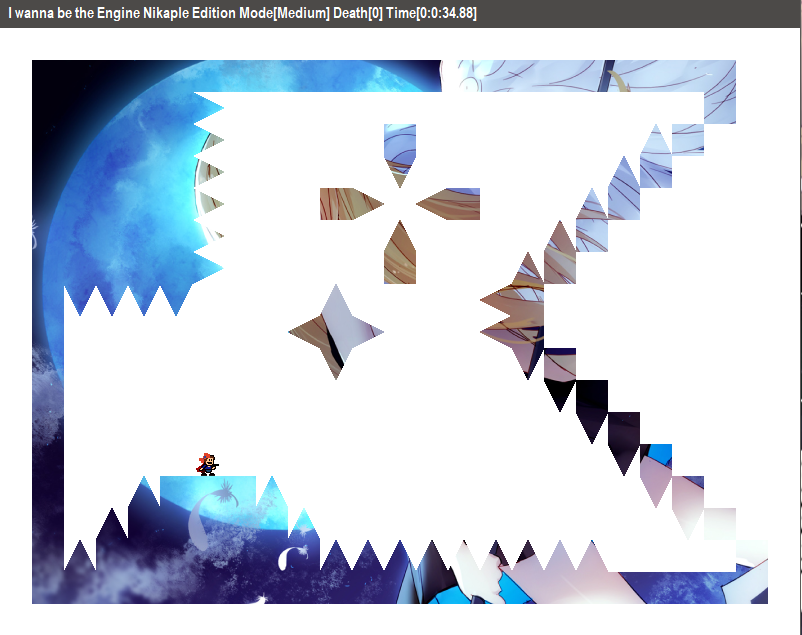Create（创建）事件：

```1 2 surf1 = surface_create(room_width, room_height); surf2 = surface_create(room_width, room_height); ```

End Step（步结束）事件：

```1 2 3 4 5 6 7 8 9 10 11 12 13 14 15 16 17 18 19 if (!surface_exists(surf1)) surf1 = surface_create(room_width, room_height); if (!surface_exists(surf2)) surf2 = surface_create(room_width, room_height); surface_set_target(surf1); draw_clear_alpha(c_black, 0); // 下面的 with 改成要被图像覆盖的 obj with (block) drawSelf(); with (playerKiller) drawSelf(); surface_set_target(surf2); draw_clear_alpha(c_black, 0); // 下面的这个函数改成绘制你的图像，不限于绘制 background，可以绘制 sprite，绘制形状，甚至绘制其他表面 draw_background(backRay, 0, 0); draw_set_blend_mode_ext(bm_zero, bm_src_alpha); draw_surface(surf1, 0, 0); draw_set_blend_mode(bm_normal); surface_reset_target(); ```

Draw（绘制）事件：

```1 draw_surface(surf2, 0, 0); ```

Destroy（销毁）事件中：

```1 2 3 4 if (surface_exists(surf1)) surface_free(surf1); if (surface_exists(surf2)) surface_free(surf2); ```

### 放大镜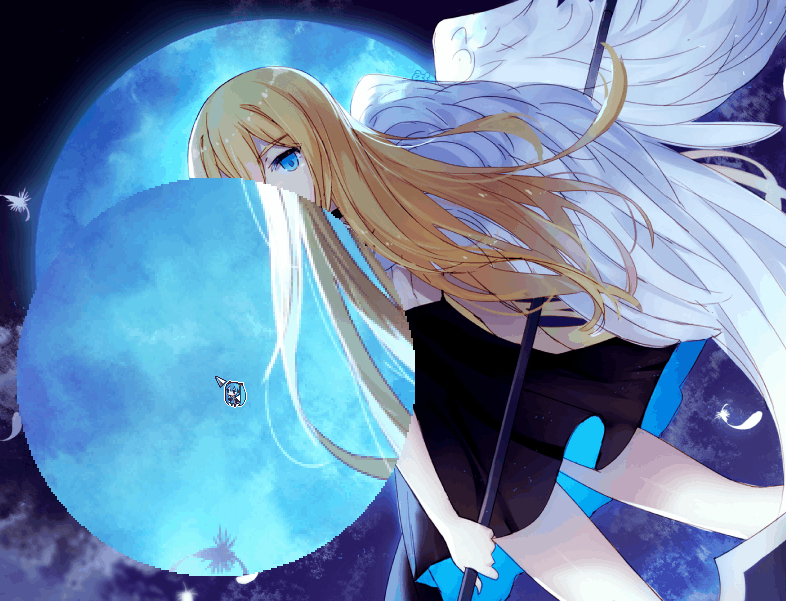Create（创建）事件：

```1 2 3 4 5 6 7 surf1 = surface_create(room_width, room_height); surf2 = surface_create(room_width, room_height); isRedraw = false; // 放大倍数 scale = 2; // 放大镜半径 radius = 100; ```

End Step（步结束）事件：

```1 2 3 4 5 6 7 8 9 10 11 12 13 14 15 16 17 18 19 20 21 22 23 24 if (!surface_exists(surf1)) surf1 = surface_create(room_width, room_height); if (!surface_exists(surf2)) surf1 = surface_create(room_width, room_height); surface_set_target(surf1); draw_clear_alpha(c_black, 0); isRedraw = true; screen_redraw(); isRedraw = false; surface_set_target(surf2); draw_clear_alpha(c_black, 0); draw_set_circle_precision(64); draw_set_color(c_black); draw_set_alpha(1); draw_circle(mouse_x, mouse_y, radius, 0); draw_set_blend_mode_ext(bm_dest_alpha, bm_inv_src_alpha); draw_surface(surf1, 0, 0); draw_set_blend_mode(bm_normal); draw_set_color(c_white); surface_reset_target(); ```

Draw（绘制）事件：

```1 2 if (!isRedraw) draw_surface_ext(surf2, mouse_x * (1 - scale), mouse_y * (1 - scale), scale, scale, 0, c_white, 1); ```

Destroy（销毁）事件：

```1 2 3 4 if (surface_exists(surf1)) surface_free(surf1); if (surface_exists(surf2)) surface_free(surf2); ```

### 表面上的反色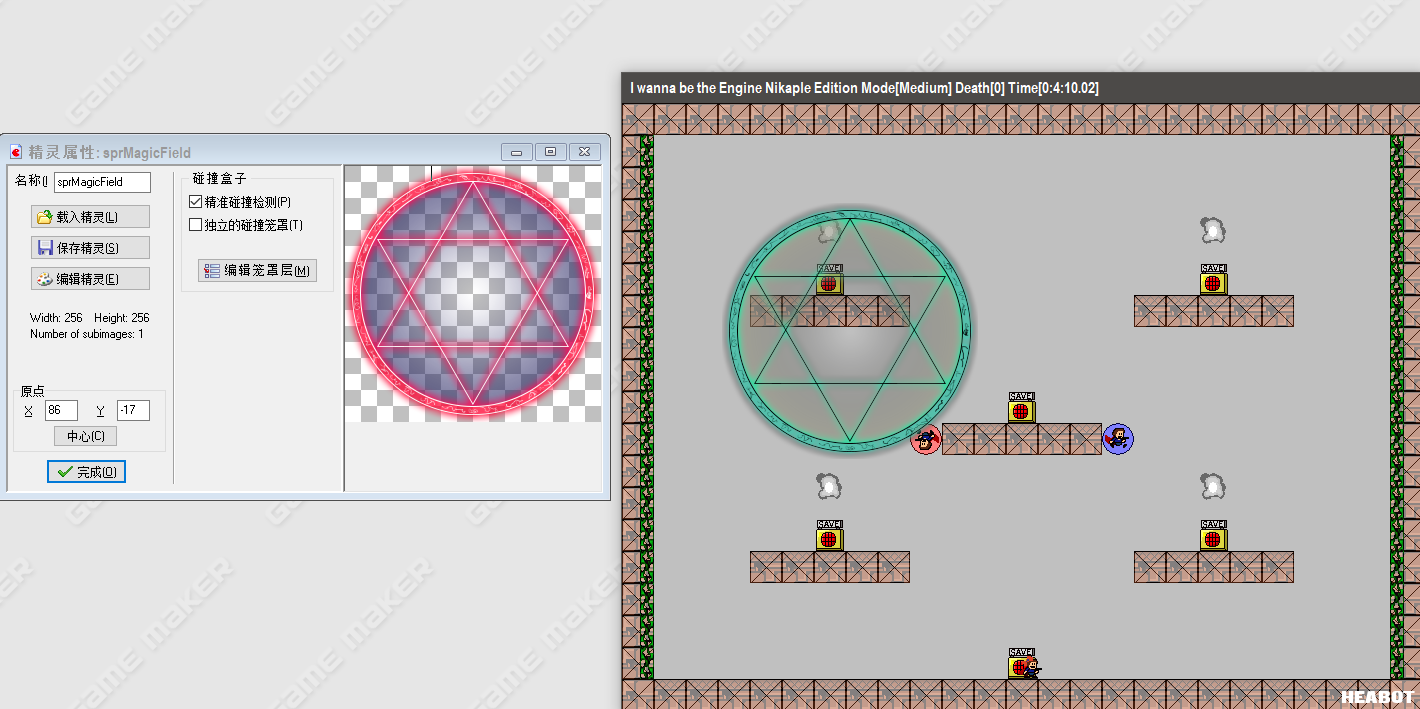Create（创建）事件：

```1 2 surf1 = surface_create(room_width, room_height); surf2 = surface_create(room_width, room_height); ```

End Step（步结束）事件：

```1 2 3 4 5 6 7 8 9 10 11 12 13 14 15 16 17 18 19 20 21 22 23 24 25 26 27 28 if (!surface_exists(surf1)) surf1 = surface_create(room_width, room_height); if (!surface_exists(surf2)) surf1 = surface_create(room_width, room_height); surface_set_target(surf1); draw_clear_alpha(0, 0); // 此处绘制任何你想要反色的精灵，背景图片，表面。 draw_sprite(sprMagicField, 0, 100, 100); draw_set_blend_mode_ext(bm_inv_dest_color, bm_zero); draw_set_color(c_white); draw_set_alpha(1); draw_rectangle(0, 0, room_width, room_height, false); draw_set_blend_mode(bm_normal); surface_set_target(surf2); draw_clear_alpha(0, 0); draw_set_blend_mode_ext(bm_one, bm_zero); draw_surface_ext(surf1, 0, 0, 1, 1, 0, c_white, 0); surface_set_target(surf1); draw_clear_alpha(0, 0); // 此处再次绘制你想要反色的图像以获取其透明度，但是你必须使用 draw_xxx_ext 并且设置 col 参数为 c_black。 draw_sprite_ext(sprMagicField, 0, 100, 100, 1, 1, 0, c_black, 1); draw_set_blend_mode_ext(bm_dest_alpha, bm_inv_src_alpha); draw_surface(surf2, 0, 0); draw_set_blend_mode(bm_normal); surface_reset_target(); ```

Draw（绘制）事件：

```1 draw_surface(surf1, 0, 0); ```

Destroy（销毁）事件：

```1 2 3 4 if (surface_exists(surf1)) surface_free(surf1); if (surface_exists(surf2)) surface_free(surf2); ```

## bm_normal 对表面的腐蚀

```1 2 3 4 Ro = Rs * As + Rd * (1 - As) Go = Gs * As + Gd * (1 - As) Bo = Bs * As + Bd * (1 - As) Ao = As * As + Ad * (1 - As) ```

```1 2 3 4 Ro = Rs * Ad + Rd * (1 - As) Go = Gs * Ad + Gd * (1 - As) Bo = Bs * Ad + Bd * (1 - As) Ao = As * Ad + Ad * (1 - As) ```

```1 2 3 4 Ro = Rs * 1 + 0 * (1 - As) = Rs Go = Gs * 1 + 0 * (1 - As) = Gs Bo = Bs * 1 + 0 * (1 - As) = Bs Ao = As * 1 + 1 * (1 - As) = 1 ```

```1 2 3 4 Ro = Rs * 0 + 0 * (1 - As) = 0 Go = Gs * 0 + 0 * (1 - As) = 0 Bo = Bs * 0 + 0 * (1 - As) = 0 Ao = As * 0 + 0 * (1 - As) = 0 ```